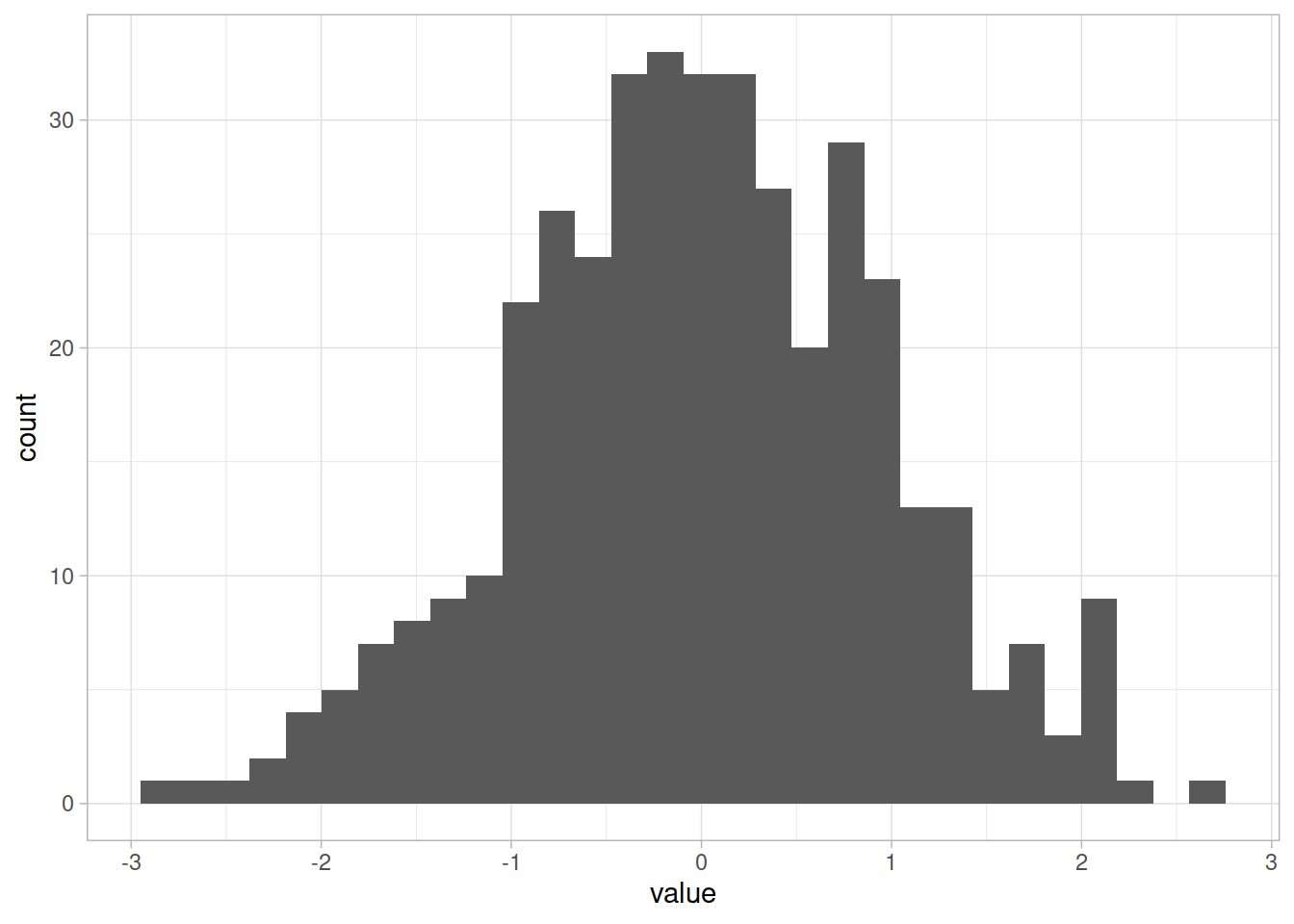You can change the default global theme (background color, grid lines etc. all non-data display):

``````# go back to a previous plot
p <- ggplot(data=df_long, mapping=aes(x=value)) + geom_histogram()

# Try different themes
p + theme_bw()``````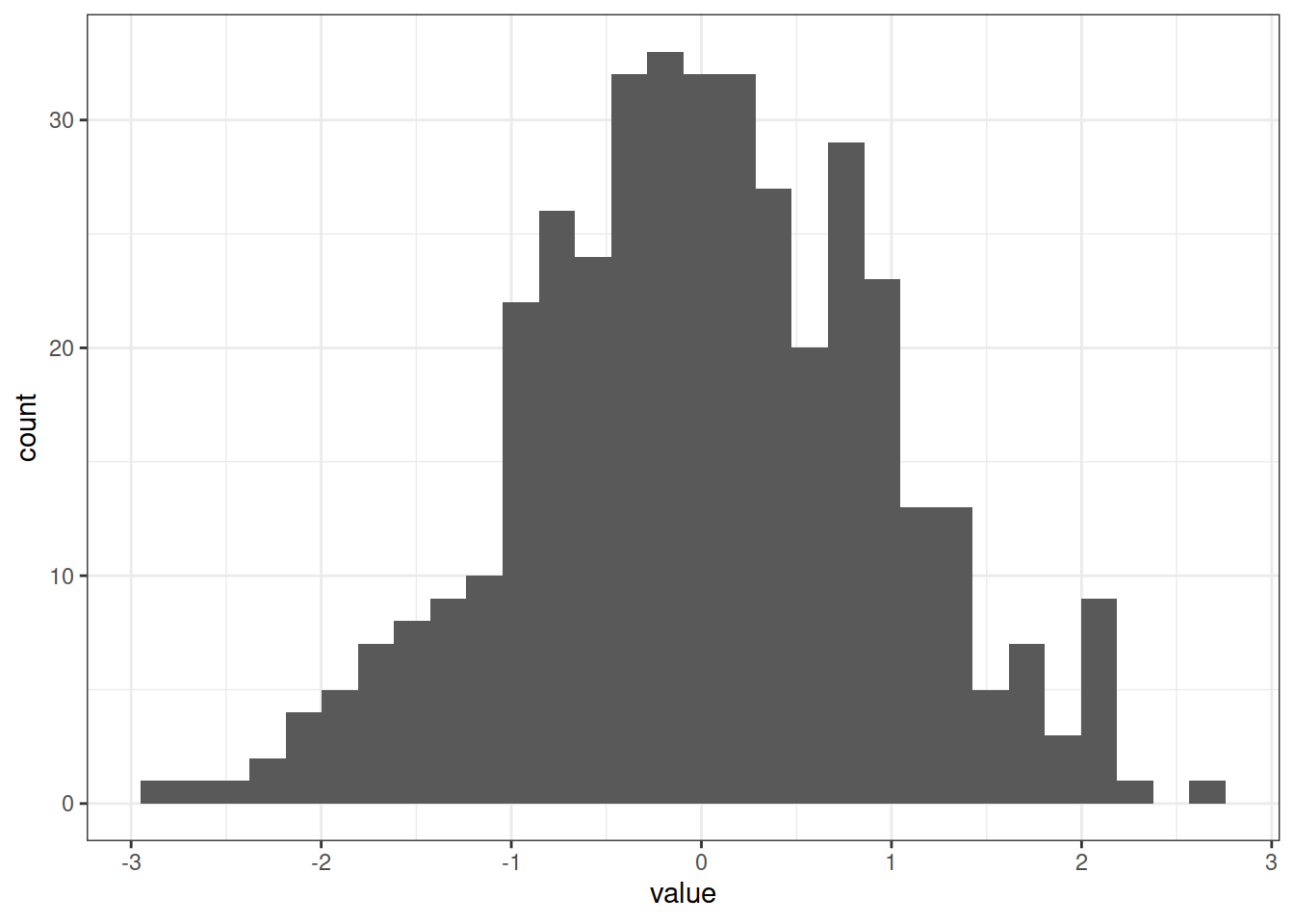``p + theme_minimal()``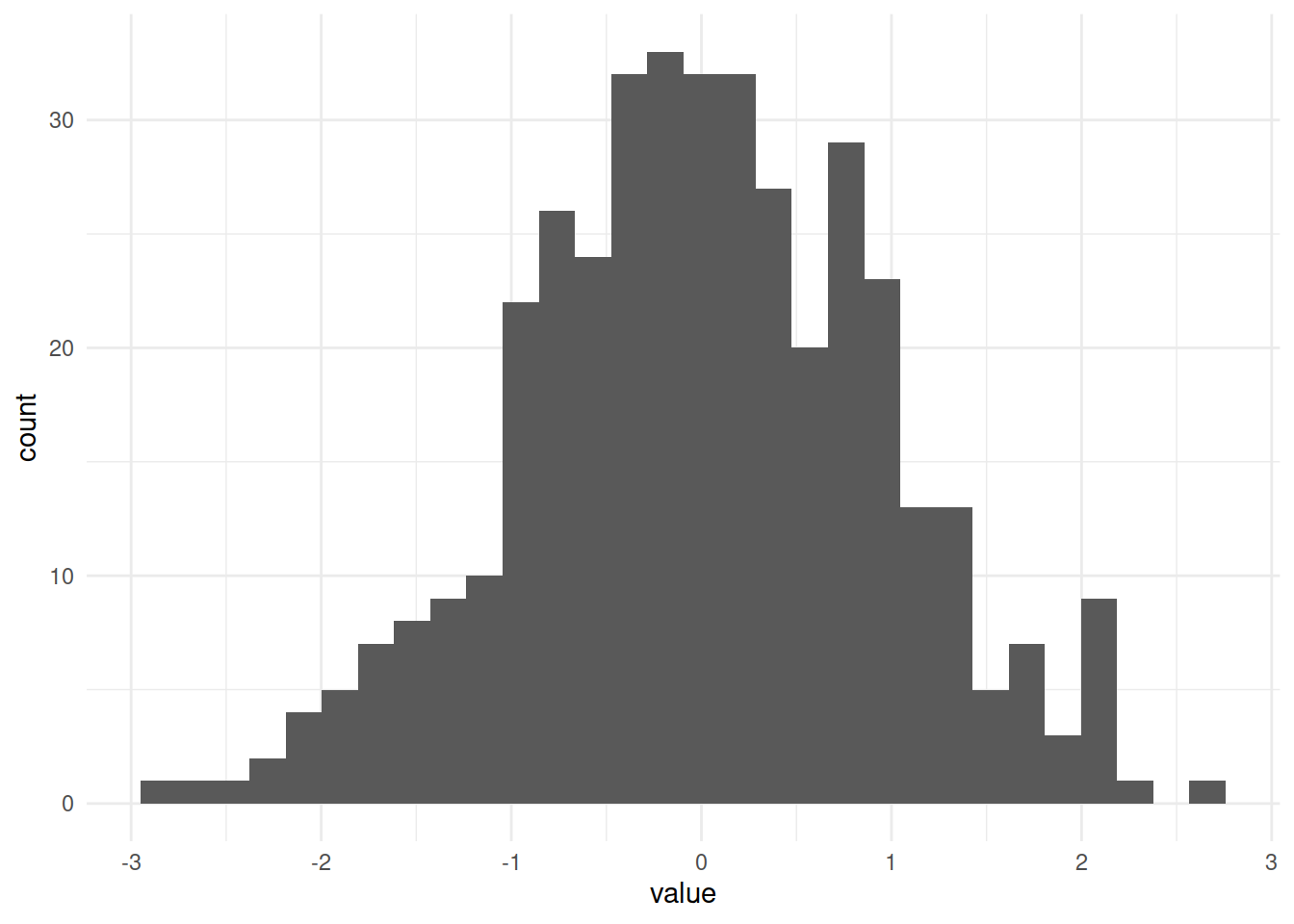``p + theme_void()``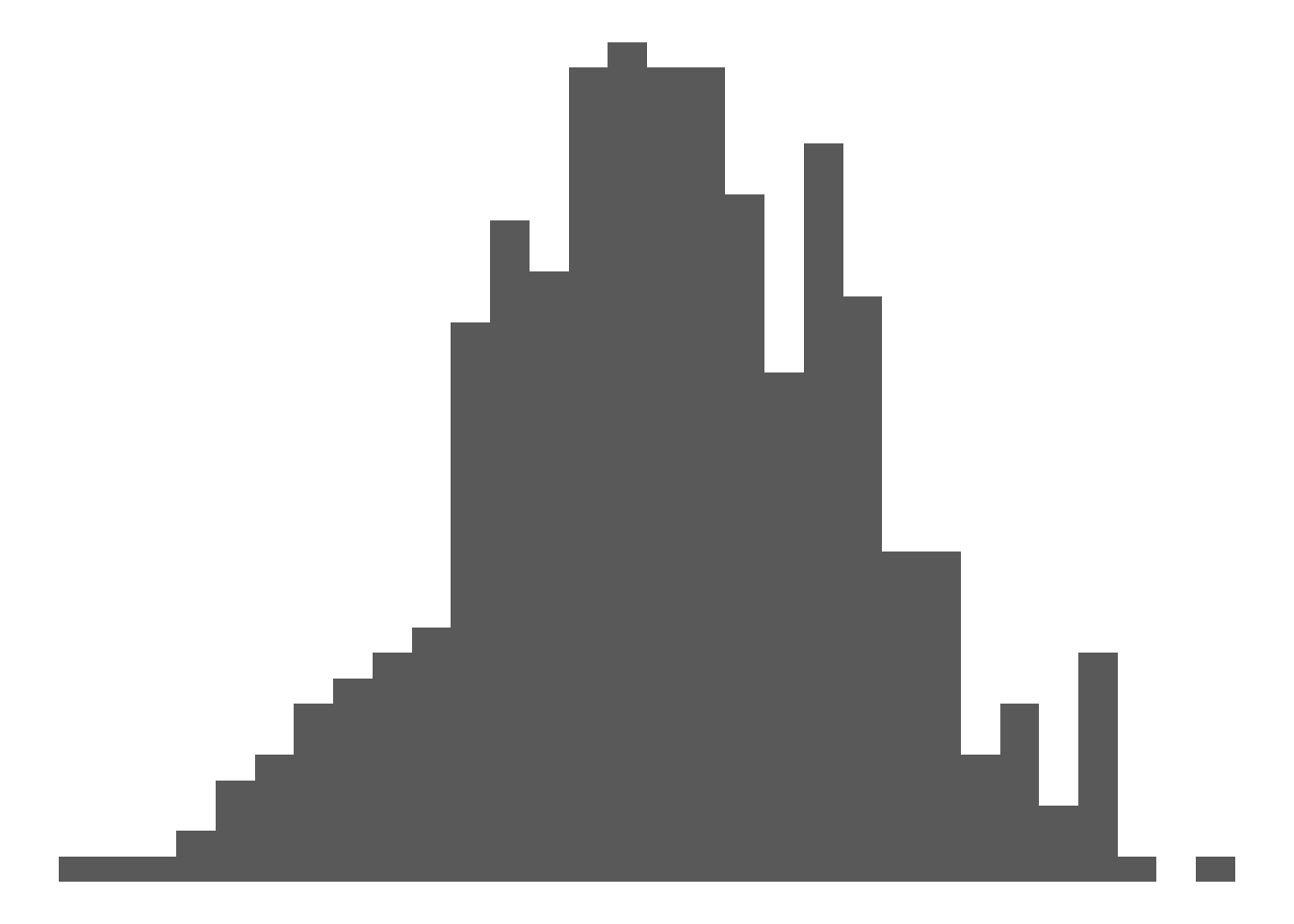``p + theme_grey()``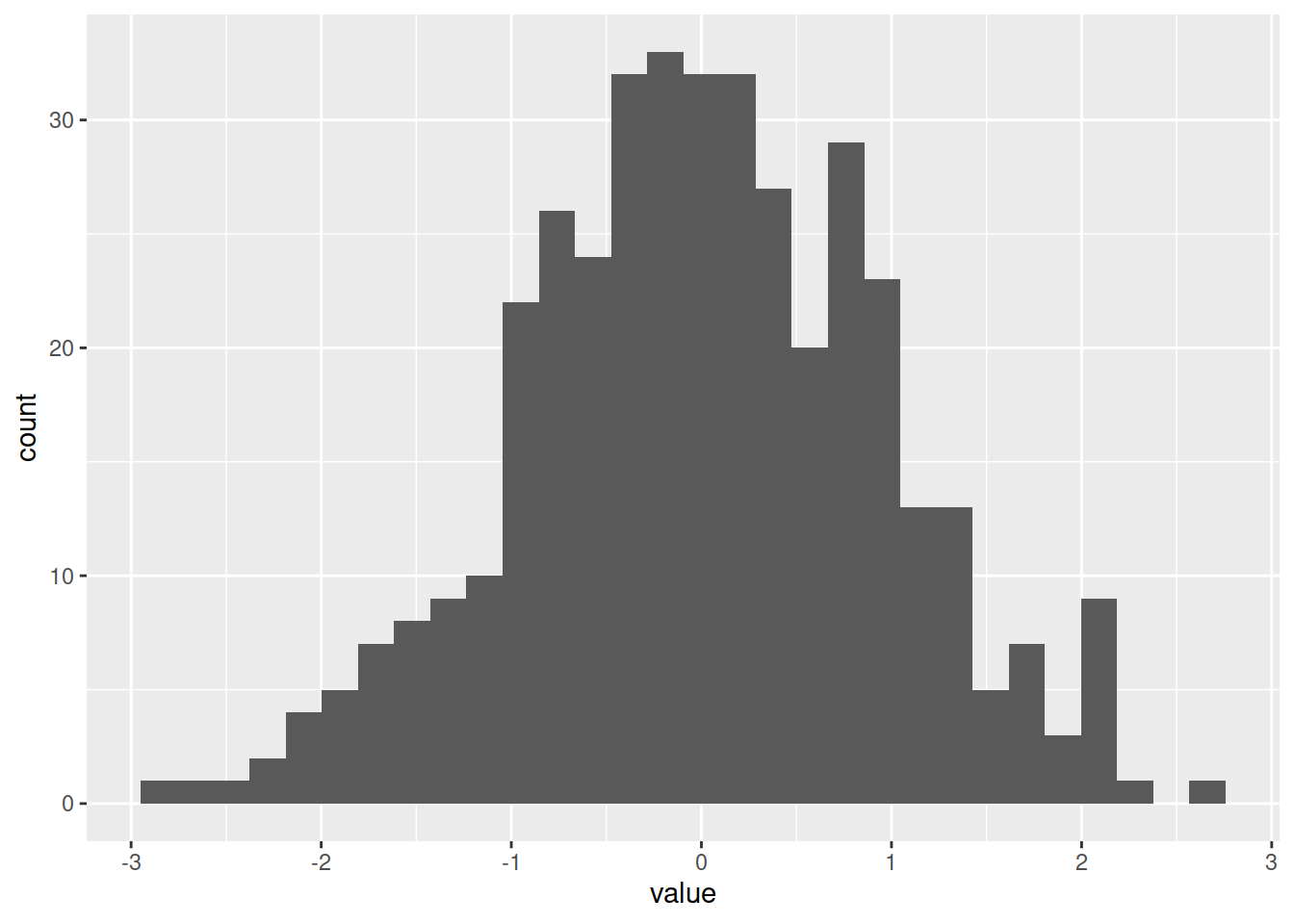``p + theme_dark()``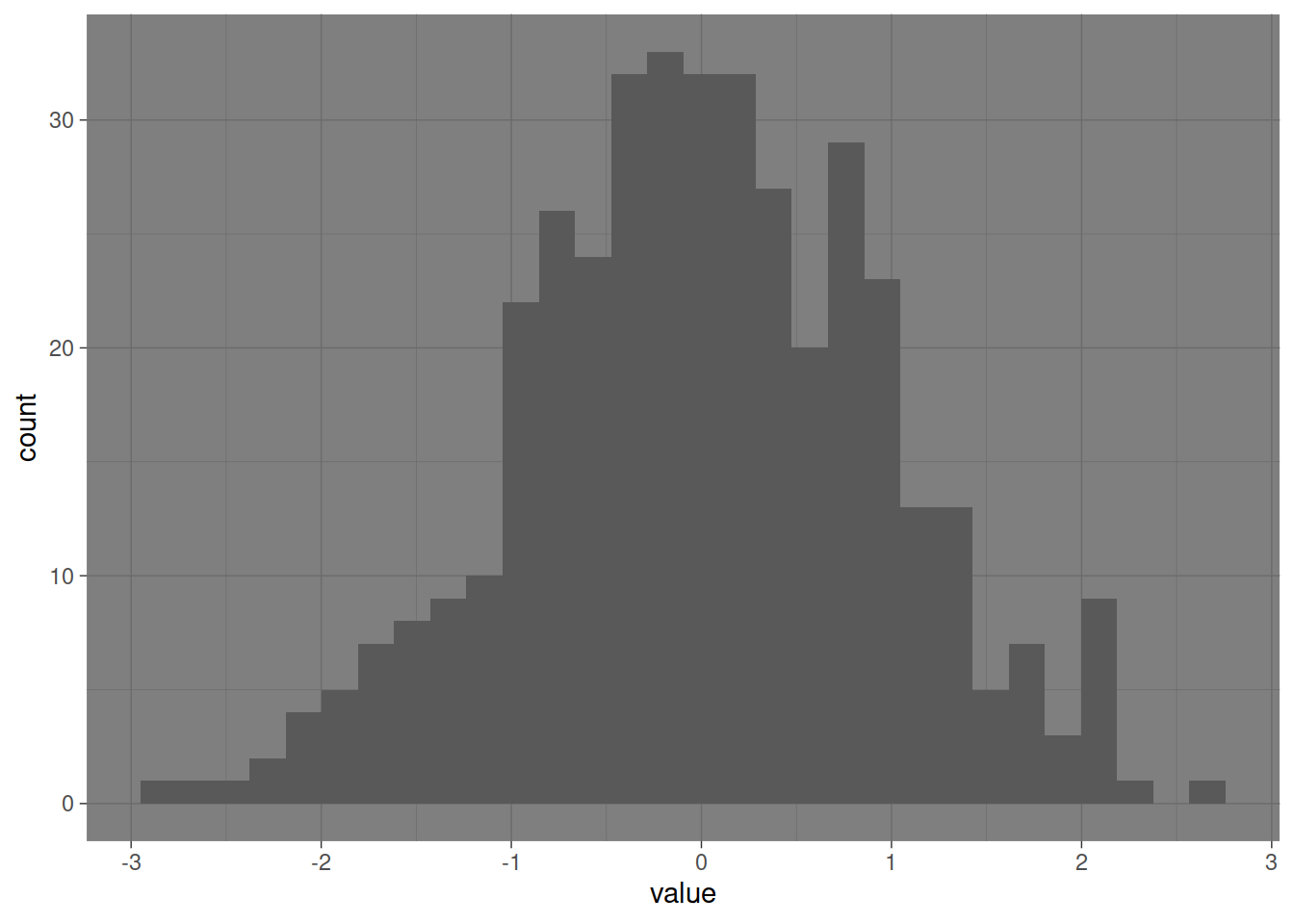``p + theme_light() ``